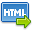Volume No. :   9

Issue No. :  1

Year :  2017

ISSN Print :  0975-4393

ISSN Online :  2349-2988Registration

## Applications of Mathematics in Various Economic Fields

 Address: Minakshi Department of Economics, Govt. College Barotiwala, Distt. Solan H.P. *Corresponding Author
DOI No: 10.5958/2349-2988.2017.00029.8

ABSTRACT:
This Paper describes the use of mathematics in various economic fields. Economics is incomplete without the use of mathematics. Mathematics and Economics go hand in hand. Economists use various mathematical tools for analyzing different economic theories. In research, we make use of mathematical equations to a large extent. Econometrics is an amalgamation of economic theory, mathematical economics, economic statistics, and mathematical statistics. Econometrics is mainly interested in empirical verification of economic theory. Econometrician uses the mathematical equations proposed by the mathematical economists but put these equations in such a form that they lend themselves to empirical testing. In research various mathematical tools are applied in analyzing different theories. Various Statistical tools as co-relation, average, t-test, Cai-square test and probable error and regression etc. are widely used. This paper gives an idea how mathematics is helpful in applying economics in solving various economic problems of an economy.
KEYWORDS:
Applications of Mathematics in Various Economic Fields.
Cite:
Minakshi. Applications of Mathematics in Various Economic Fields. Research J. Science and Tech. 2017; 9(1):175-178.[View HTML][View PDF]

Visitor's No. :   217828